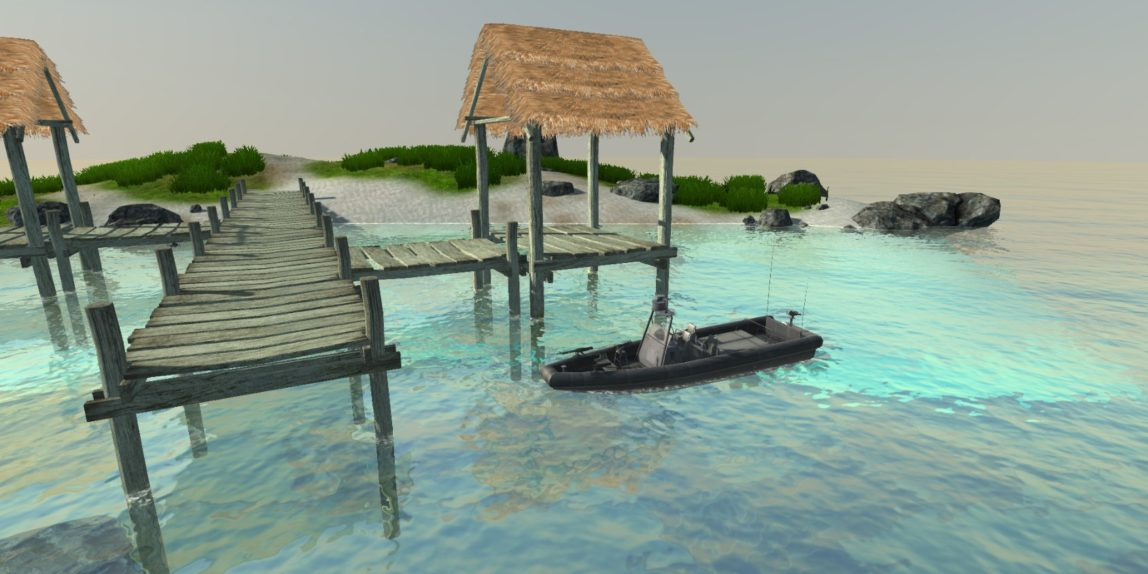# Physics: Boat## Introduction

The goal of this project was to make a boat physic using Unity rigid-bodies and C#. Note that the boat physic can be used for simple buoyancy and any types of boats with some changes in the settings.

The method that I used was inspired from this very nice series of article on Gamasutra from a senior software engineer at Avalanche Studios. The method is based on the principle that every mesh in a video game is composed of triangles. We can then determine how much triangles are above or under water. This will be the base of the algorithm

## Algorithm

Before starting anything with our boat we need to have a function that gives us the height of the water at a given point. Ideally this function is detached from the boat so we can swap it very easily if the water simulation is changed.

Now that we can know the height of the water we will iterate through the triangles composing the boat to create 2 submesh one is the underwater mesh and the other is the above water. For each triangle we will check the height of each vertices from the water. We will then check how many vertices are under water.
If 0 vertices are under water then the triangle is above the water we can add it to the above water mesh.
If 1 vertex is under water we will cut the triangle into 3 sub triangles one is under water and the other 2 are above. In the figure below the under water triangle will be JM L JH. And the two above water are : H JM JH and M JM H
Note that all of this sub triangles are added to their corresponding above or under water mesh.

If 2 vertices are under water the we will do the same thing as above except that 2 sub triangles will be under water and 1 above.

Now that we have our mesh cut in 2 parts (one above water one under) we can use them to calculate the forces on each triangles.
For the under water mesh we will calculate on each triangles :
– The Buoyancy
– The Viscous Water Resistance
– The Pressure Drag Force
– The Slamming Forces
I will not go into the details on how to calculate them but if you are interested the part 2 of the article on gamasutra explain everything.

For the above water mesh we calculate only the air resistance aka the air Drag force.

## Performances

With this algorithm I have a pretty nice result but you are maybe wondering that I iterate 2 times on each triangles of the mesh and knowing that some meshes can have thousands and thousands of triangles it may be a bit slow ? Here is a little trick to have good performance while using high poly asset. The trick is to have 2 meshes one for display and one for the boat physics. The last one is obviously hidden and is a low poly shape of the first one. For me here what I’ve got